Next: INTRODUCTION Up: No Title Previous: THE AUTHOR'S CONTRIBUTION

# LIST OF SYMBOLS AND ABBREVIATIONS

 ASSOM Adaptive-subspace self-organizing map DSS Decision support system EEG Electroencephalogram EM Expectation maximization GNP Gross national product GTM Generative topographic mapping IR Information retrieval KDD Knowledge discovery in databases MDS Multidimensional scaling PCA Principal component analysis RBF Radial basis function SOM Self-organizing map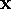,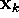input vector (data item), kth input vector t discrete time index N number of input vectors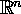input space; n-dimensional Euclidean space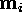ith cluster centroid, ith model vector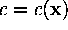index of the centroid (or model vector) that is closest toK number of cluster centroids (and reference vectors)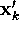projection ofd(k,l) distance betweenand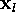d'(k,l) distance betweenand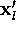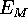cost function of the metric MDS method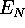cost function of the nonmetric MDS method f a monotonically increasing function used in nonmetric MDS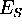cost function of Sammon's mapping q inherent dimensionality of the data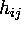neighborhood kernel in the SOM algorithm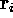location of the ith map unit on the map gridprobability density function of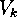Voronoi-region corresponding to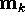, viz. the set consisting of those x for which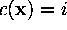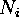number of data items in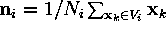centroid of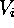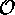computational complexity (``of the order of'')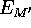a modified cost function of the metric MDS method F a decreasing weighting functionNext: INTRODUCTION Up: No Title Previous: THE AUTHOR'S CONTRIBUTION